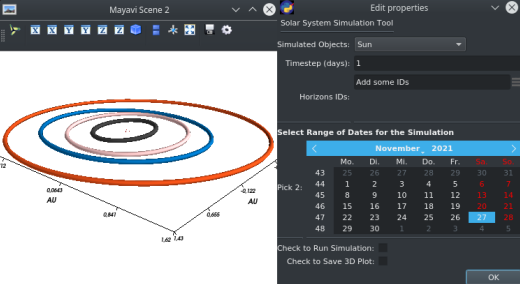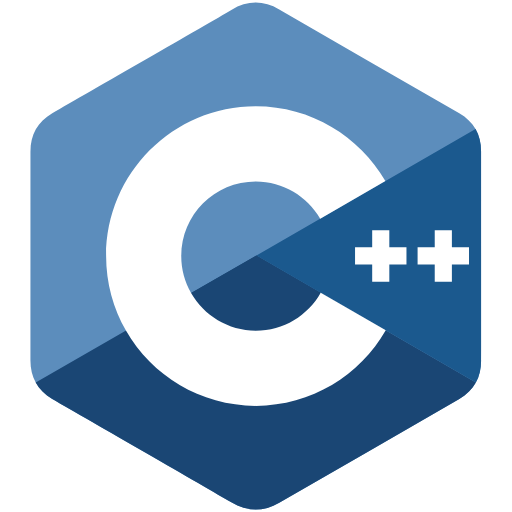# Python Programs

Title Preview Link Requirements Description Type
AstroCamREQUIREMENTS.txt A Raspberry Pi HQ Camera App written in Python using the PiCamera package to interface with the official 12Mp Sony IMX477 HQ camera for the Raspberry Pi. GUI Program
Solar System Simulation ToolboxREQUIREMENTS.txt A Solar System Simulation Tool using NASA Horizons Data to simulate the orbits in the solar system of an arbitrary selection of bodies using a Newtonian N-Body Integrator. GUI Program

# C++ Scripts

Pi CalculatorNone Script to calculate the value of Pi according to the Leibniz formula with an arbitrary input for the amount of iterations k Numerical Script
Square Function Root SeekerNone Script to calculate the root between the input boundaries x1 and x2 of any square function in the shape ax^2+bx+c. It works according to the bisection method up to a search depth of tol = 1e-6. Numerical Script
Numerical IntegratorNone Script to calculate the integral between the input boundaries x1 and x2 of any square function in the shape ax^2+bx+c. It uses the Boode-Bole-Mint rule up to a user-defined number of intervals n. Numerical Script